# Relative Valuation Aswath Damodaran 85 Why relative valuation

• Slides: 95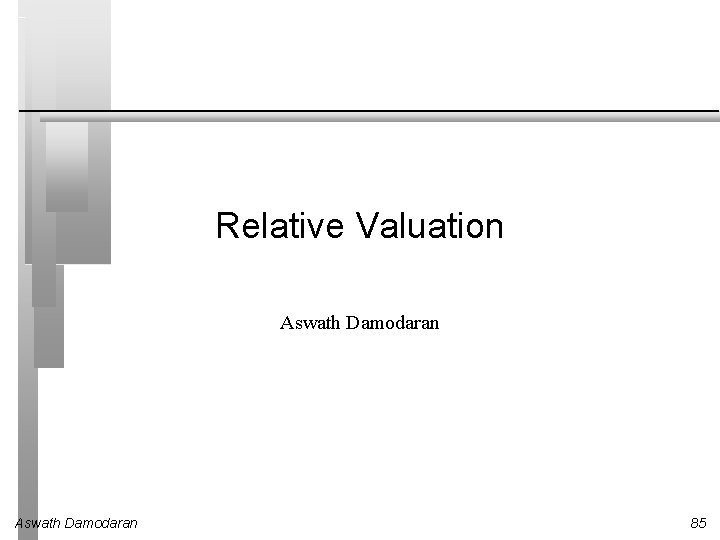Relative Valuation Aswath Damodaran 85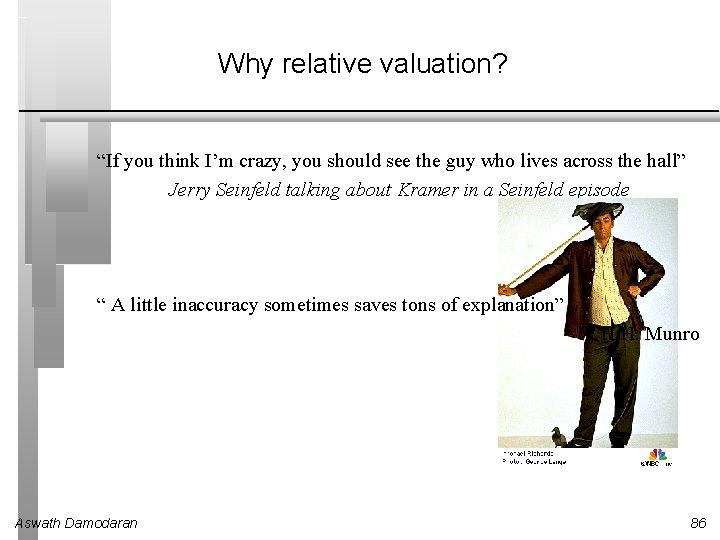Why relative valuation? “If you think I’m crazy, you should see the guy who lives across the hall” Jerry Seinfeld talking about Kramer in a Seinfeld episode “ A little inaccuracy sometimes saves tons of explanation” H. H. Munro Aswath Damodaran 86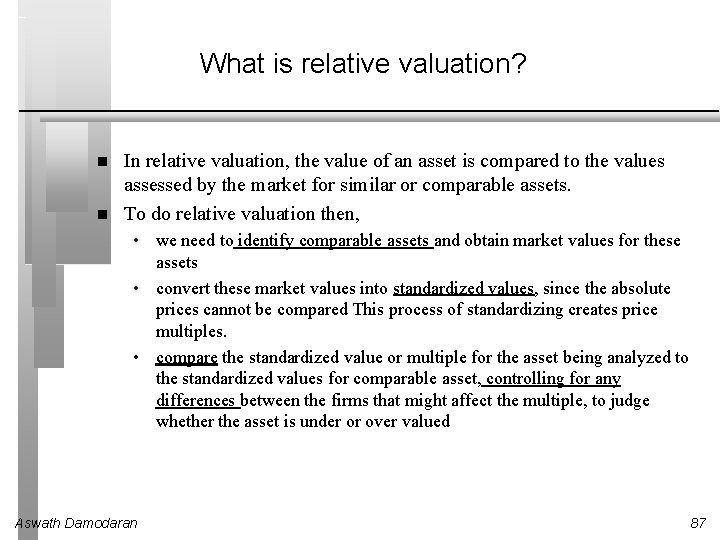What is relative valuation? In relative valuation, the value of an asset is compared to the values assessed by the market for similar or comparable assets. To do relative valuation then, • we need to identify comparable assets and obtain market values for these assets • convert these market values into standardized values, since the absolute prices cannot be compared This process of standardizing creates price multiples. • compare the standardized value or multiple for the asset being analyzed to the standardized values for comparable asset, controlling for any differences between the firms that might affect the multiple, to judge whether the asset is under or over valued Aswath Damodaran 87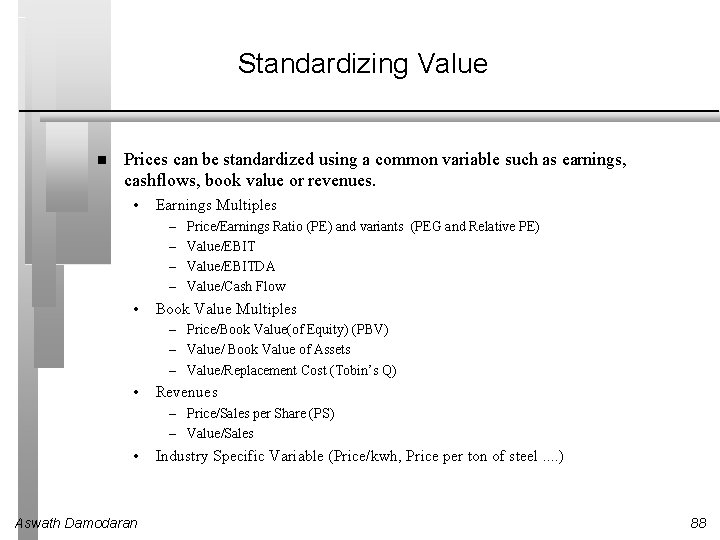Standardizing Value Prices can be standardized using a common variable such as earnings, cashflows, book value or revenues. • Earnings Multiples – – • Price/Earnings Ratio (PE) and variants (PEG and Relative PE) Value/EBITDA Value/Cash Flow Book Value Multiples – Price/Book Value(of Equity) (PBV) – Value/ Book Value of Assets – Value/Replacement Cost (Tobin’s Q) • Revenues – Price/Sales per Share (PS) – Value/Sales • Aswath Damodaran Industry Specific Variable (Price/kwh, Price per ton of steel. . ) 88The Four Steps to Understanding Multiples Define the multiple • Describe the multiple • Too many people who use a multiple have no idea what its cross sectional distribution is. If you do not know what the cross sectional distribution of a multiple is, it is difficult to look at a number and pass judgment on whether it is too high or low. Analyze the multiple • In use, the same multiple can be defined in different ways by different users. When comparing and using multiples, estimated by someone else, it is critical that we understand how the multiples have been estimated It is critical that we understand the fundamentals that drive each multiple, and the nature of the relationship between the multiple and each variable. Apply the multiple • Aswath Damodaran Defining the comparable universe and controlling for differences is far more difficult in practice than it is in theory. 89Definitional Tests Is the multiple consistently defined? • Proposition 1: Both the value (the numerator) and the standardizing variable ( the denominator) should be to the same claimholders in the firm. In other words, the value of equity should be divided by equity earnings or equity book value, and firm value should be divided by firm earnings or book value. Is the multiple uniformly estimated? • The variables used in defining the multiple should be estimated uniformly across assets in the “comparable firm” list. • If earnings-based multiples are used, the accounting rules to measure earnings should be applied consistently across assets. The same rule applies with book-value based multiples. Aswath Damodaran 90Descriptive Tests What is the average and standard deviation for this multiple, across the universe (market)? What is the median for this multiple? • The median for this multiple is often a more reliable comparison point. How large are the outliers to the distribution, and how do we deal with the outliers? • Throwing out the outliers may seem like an obvious solution, but if the outliers all lie on one side of the distribution (they usually are large positive numbers), this can lead to a biased estimate. Are there cases where the multiple cannot be estimated? Will ignoring these cases lead to a biased estimate of the multiple? How has this multiple changed over time? Aswath Damodaran 91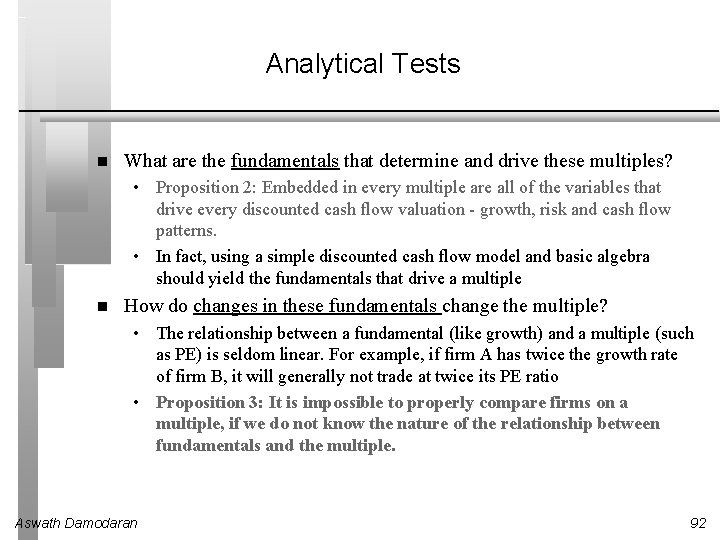Analytical Tests What are the fundamentals that determine and drive these multiples? • Proposition 2: Embedded in every multiple are all of the variables that drive every discounted cash flow valuation - growth, risk and cash flow patterns. • In fact, using a simple discounted cash flow model and basic algebra should yield the fundamentals that drive a multiple How do changes in these fundamentals change the multiple? • The relationship between a fundamental (like growth) and a multiple (such as PE) is seldom linear. For example, if firm A has twice the growth rate of firm B, it will generally not trade at twice its PE ratio • Proposition 3: It is impossible to properly compare firms on a multiple, if we do not know the nature of the relationship between fundamentals and the multiple. Aswath Damodaran 92Application Tests Given the firm that we are valuing, what is a “comparable” firm? • While traditional analysis is built on the premise that firms in the same sector are comparable firms, valuation theory would suggest that a comparable firm is one which is similar to the one being analyzed in terms of fundamentals. • Proposition 4: There is no reason why a firm cannot be compared with another firm in a very different business, if the two firms have the same risk, growth and cash flow characteristics. Given the comparable firms, how do we adjust for differences across firms on the fundamentals? • Proposition 5: It is impossible to find an exactly identical firm to the one you are valuing. Aswath Damodaran 93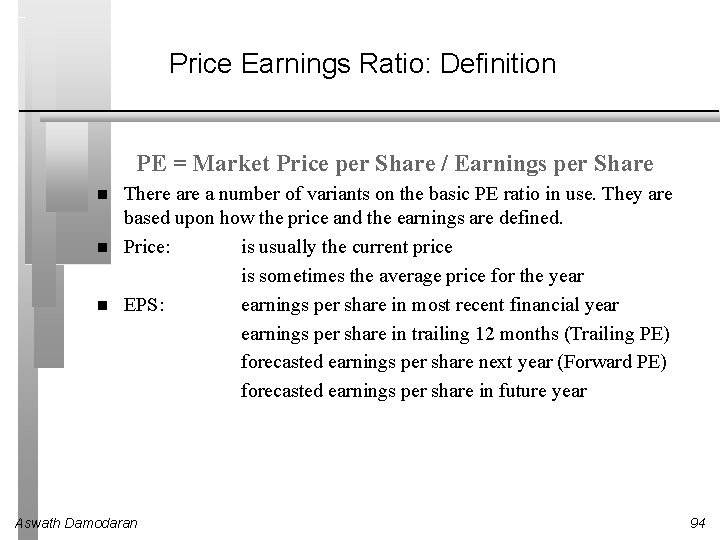Price Earnings Ratio: Definition PE = Market Price per Share / Earnings per Share There a number of variants on the basic PE ratio in use. They are based upon how the price and the earnings are defined. Price: is usually the current price is sometimes the average price for the year EPS: earnings per share in most recent financial year earnings per share in trailing 12 months (Trailing PE) forecasted earnings per share next year (Forward PE) forecasted earnings per share in future year Aswath Damodaran 94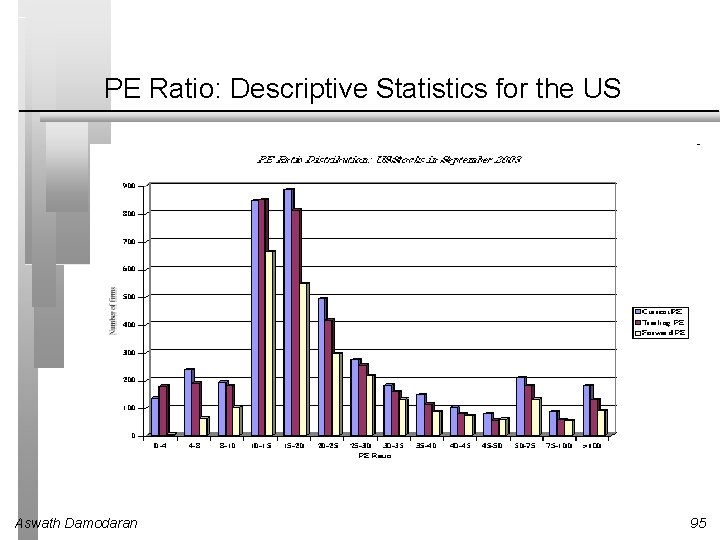PE Ratio: Descriptive Statistics for the US Aswath Damodaran 95PE: Deciphering the Distribution Aswath Damodaran 96PE Distribution: Europe and Emerging Markets in September 2003 Aswath Damodaran 97Comparing PE Ratios: US, Europe and Emerging Markets Median PE US = 18. 25 Europe = 15. 09 Em Mkts = 14. 32 Aswath Damodaran 98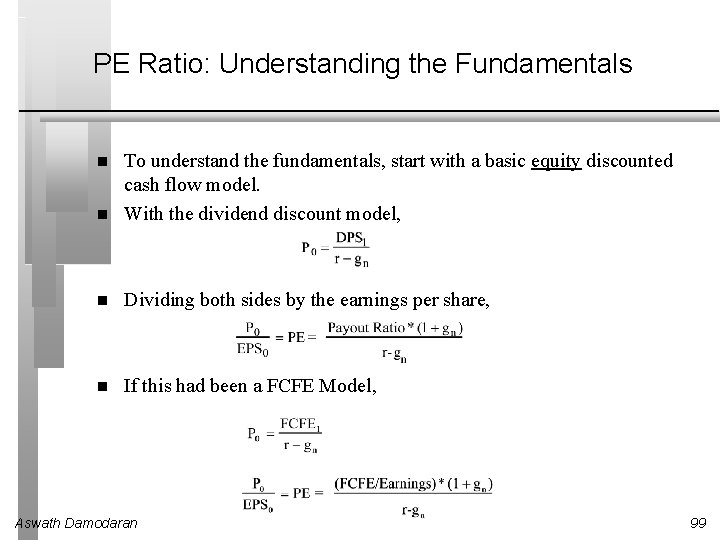PE Ratio: Understanding the Fundamentals To understand the fundamentals, start with a basic equity discounted cash flow model. With the dividend discount model, Dividing both sides by the earnings per share, If this had been a FCFE Model, Aswath Damodaran 99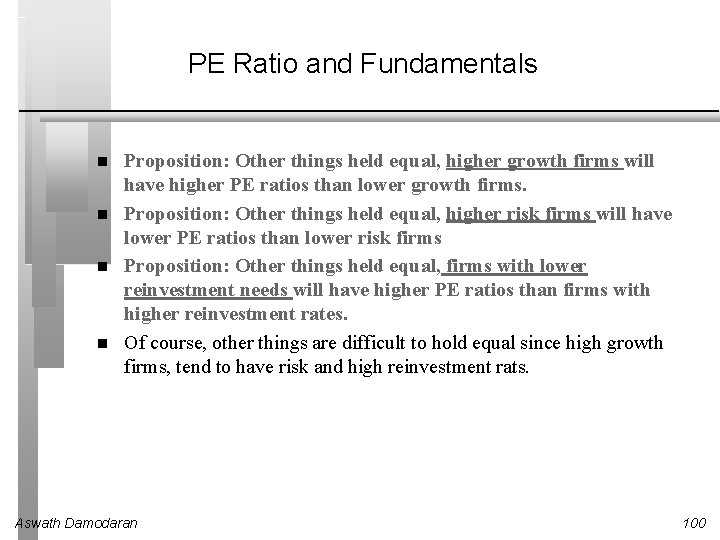PE Ratio and Fundamentals Proposition: Other things held equal, higher growth firms will have higher PE ratios than lower growth firms. Proposition: Other things held equal, higher risk firms will have lower PE ratios than lower risk firms Proposition: Other things held equal, firms with lower reinvestment needs will have higher PE ratios than firms with higher reinvestment rates. Of course, other things are difficult to hold equal since high growth firms, tend to have risk and high reinvestment rats. Aswath Damodaran 100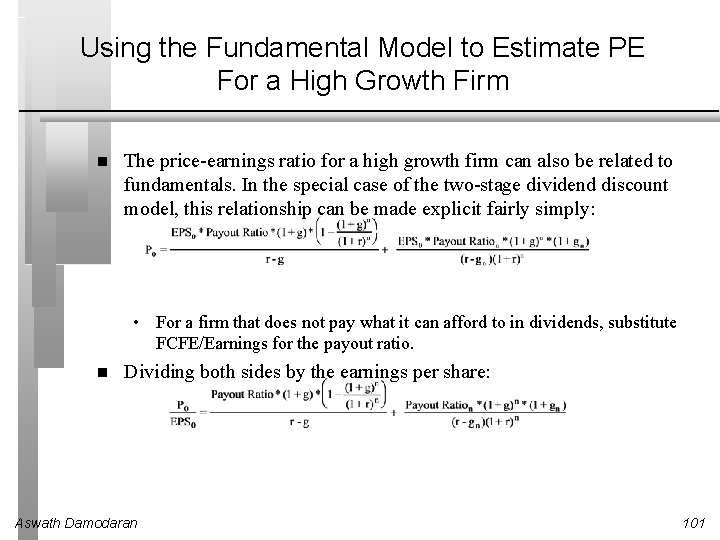Using the Fundamental Model to Estimate PE For a High Growth Firm The price-earnings ratio for a high growth firm can also be related to fundamentals. In the special case of the two-stage dividend discount model, this relationship can be made explicit fairly simply: • For a firm that does not pay what it can afford to in dividends, substitute FCFE/Earnings for the payout ratio. Dividing both sides by the earnings per share: Aswath Damodaran 101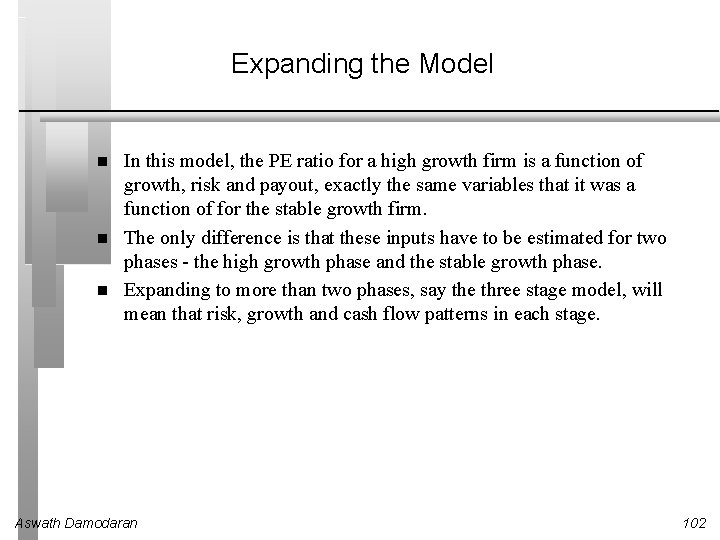Expanding the Model In this model, the PE ratio for a high growth firm is a function of growth, risk and payout, exactly the same variables that it was a function of for the stable growth firm. The only difference is that these inputs have to be estimated for two phases - the high growth phase and the stable growth phase. Expanding to more than two phases, say the three stage model, will mean that risk, growth and cash flow patterns in each stage. Aswath Damodaran 102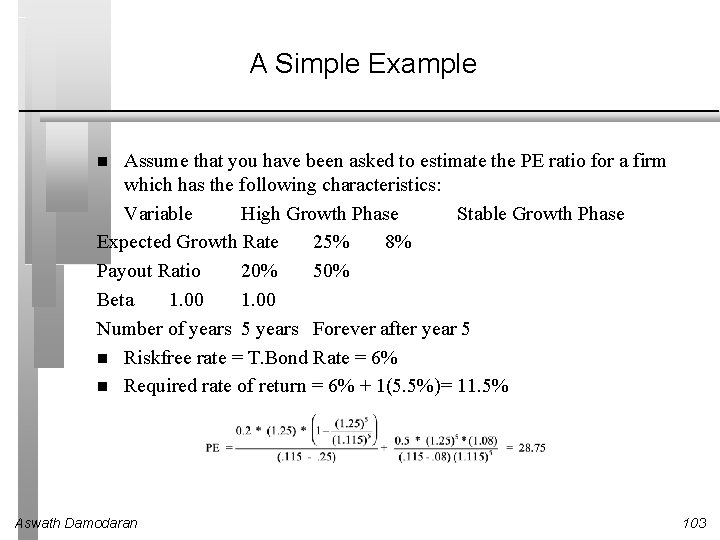A Simple Example Assume that you have been asked to estimate the PE ratio for a firm which has the following characteristics: Variable High Growth Phase Stable Growth Phase Expected Growth Rate 25% 8% Payout Ratio 20% 50% Beta 1. 00 Number of years 5 years Forever after year 5 Riskfree rate = T. Bond Rate = 6% Required rate of return = 6% + 1(5. 5%)= 11. 5% Aswath Damodaran 103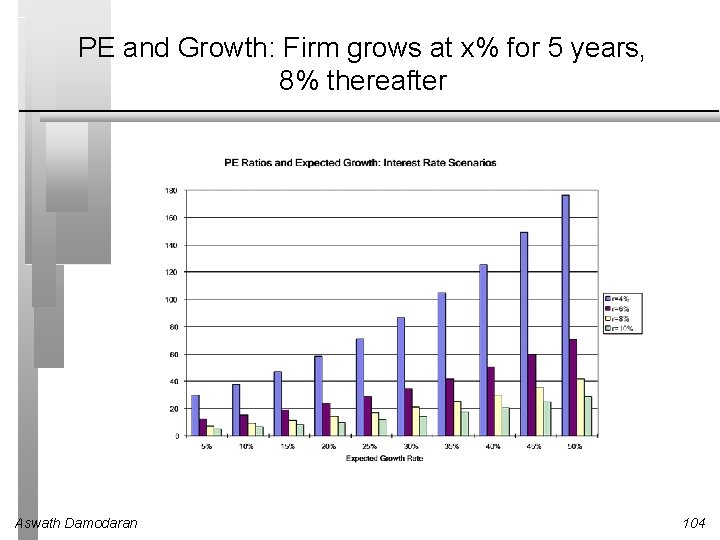PE and Growth: Firm grows at x% for 5 years, 8% thereafter Aswath Damodaran 104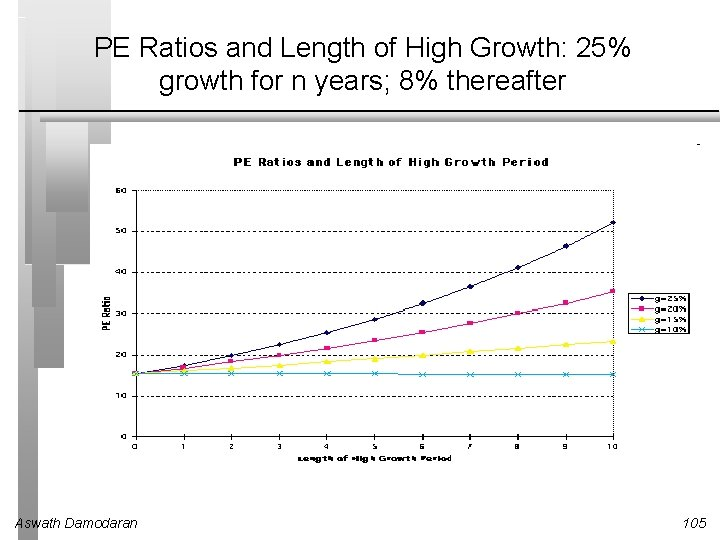PE Ratios and Length of High Growth: 25% growth for n years; 8% thereafter Aswath Damodaran 105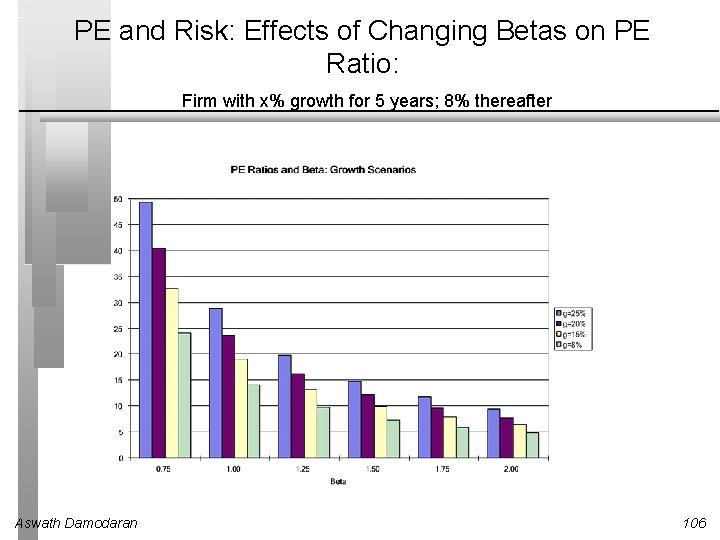PE and Risk: Effects of Changing Betas on PE Ratio: Firm with x% growth for 5 years; 8% thereafter Aswath Damodaran 106PE and Payout Aswath Damodaran 107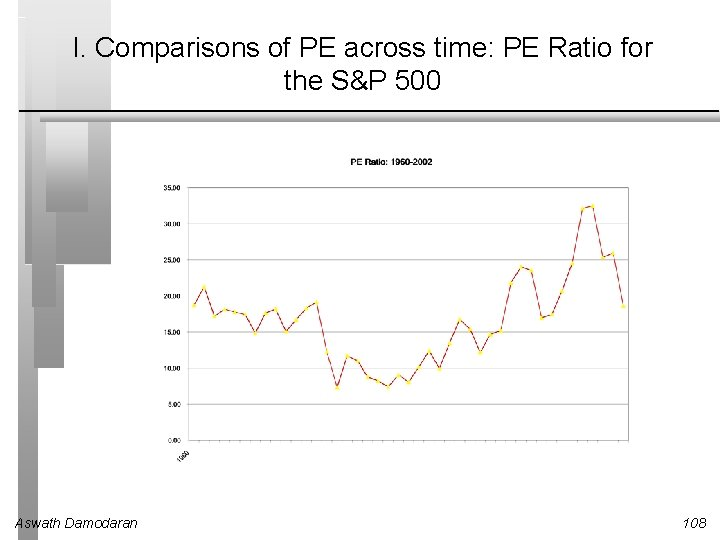I. Comparisons of PE across time: PE Ratio for the S&P 500 Aswath Damodaran 108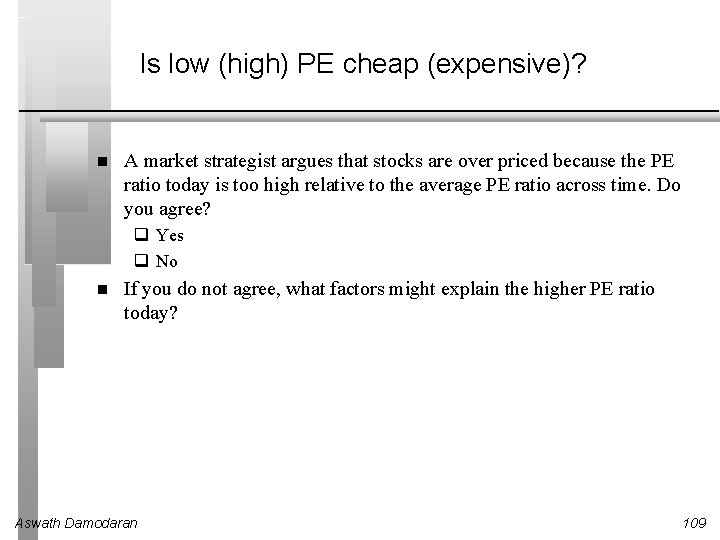Is low (high) PE cheap (expensive)? A market strategist argues that stocks are over priced because the PE ratio today is too high relative to the average PE ratio across time. Do you agree? q Yes q No If you do not agree, what factors might explain the higher PE ratio today? Aswath Damodaran 109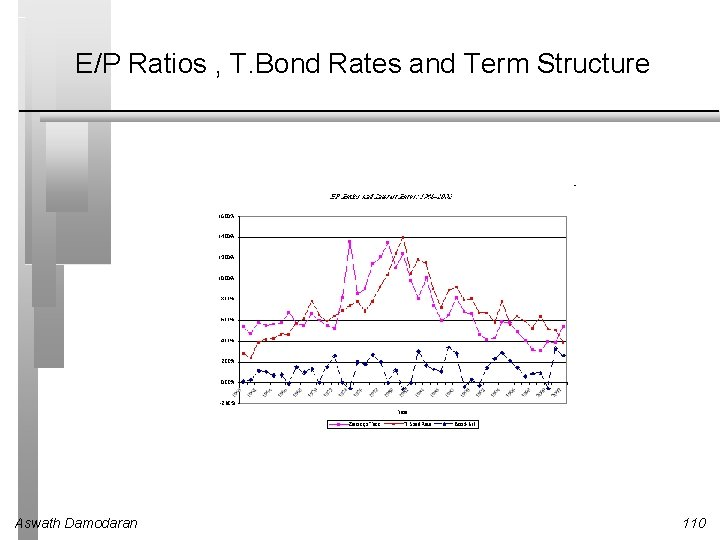E/P Ratios , T. Bond Rates and Term Structure Aswath Damodaran 110Regression Results There is a strong positive relationship between E/P ratios and T. Bond rates, as evidenced by the correlation of 0. 70 between the two variables. , In addition, there is evidence that the term structure also affects the PE ratio. In the following regression, using 1960 -2002 data, we regress E/P ratios against the level of T. Bond rates and a term structure variable (T. Bond - T. Bill rate) E/P = 1. 98% + 0. 762 T. Bond Rate - 0. 387 (T. Bond Rate-T. Bill Rate) (1. 94) (6. 29) (-1. 42) R squared = 50. 5% Aswath Damodaran 111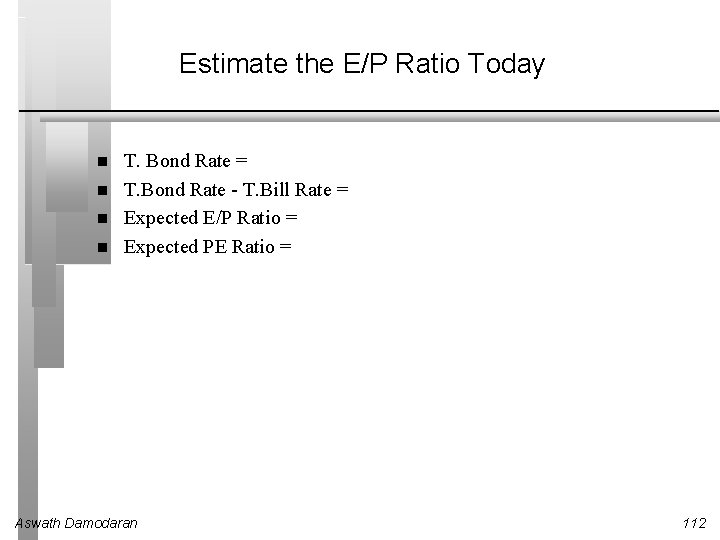Estimate the E/P Ratio Today T. Bond Rate = T. Bond Rate - T. Bill Rate = Expected E/P Ratio = Expected PE Ratio = Aswath Damodaran 112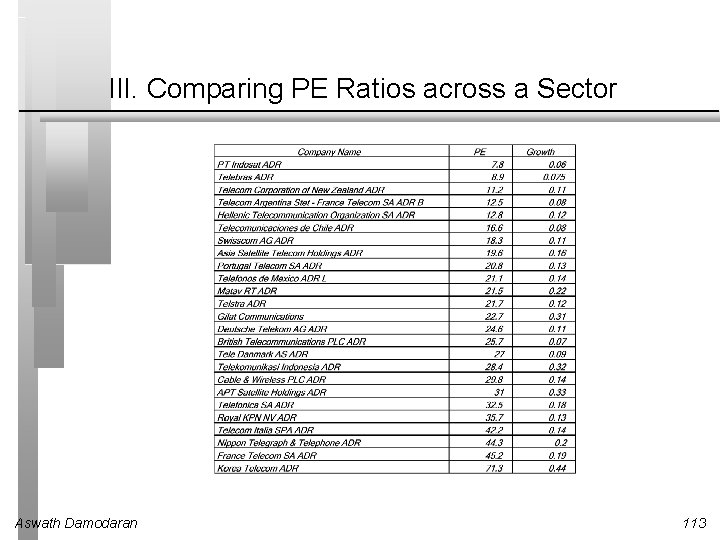III. Comparing PE Ratios across a Sector Aswath Damodaran 113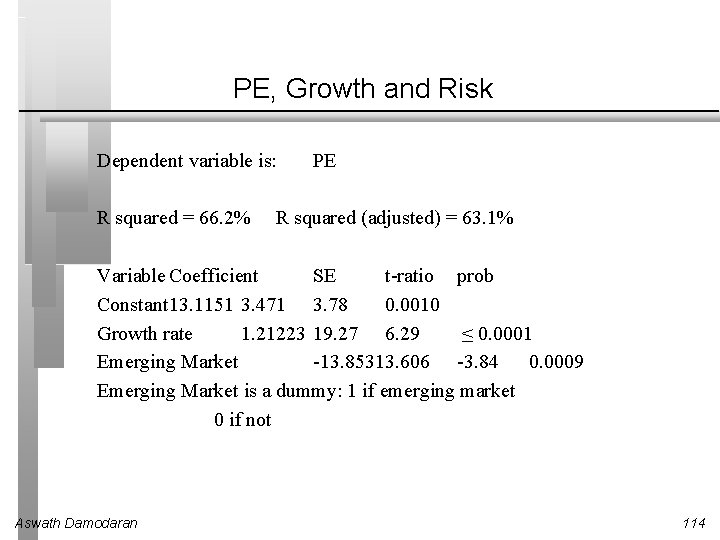PE, Growth and Risk Dependent variable is: R squared = 66. 2% PE R squared (adjusted) = 63. 1% Variable Coefficient SE t-ratio prob Constant 13. 1151 3. 471 3. 78 0. 0010 Growth rate 1. 21223 19. 27 6. 29 ≤ 0. 0001 Emerging Market -13. 85313. 606 -3. 84 0. 0009 Emerging Market is a dummy: 1 if emerging market 0 if not Aswath Damodaran 114Is Telebras under valued? Predicted PE = 13. 12 + 1. 2122 (7. 5) - 13. 85 (1) = 8. 35 At an actual price to earnings ratio of 8. 9, Telebras is slightly overvalued. Aswath Damodaran 115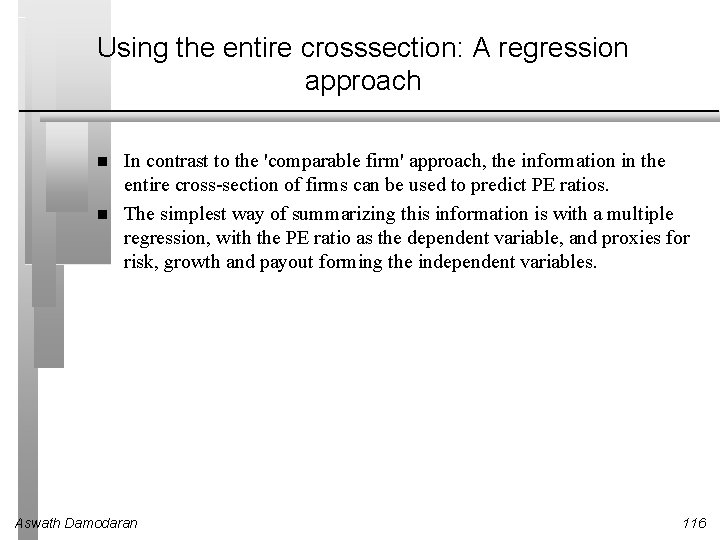Using the entire crosssection: A regression approach In contrast to the 'comparable firm' approach, the information in the entire cross-section of firms can be used to predict PE ratios. The simplest way of summarizing this information is with a multiple regression, with the PE ratio as the dependent variable, and proxies for risk, growth and payout forming the independent variables. Aswath Damodaran 116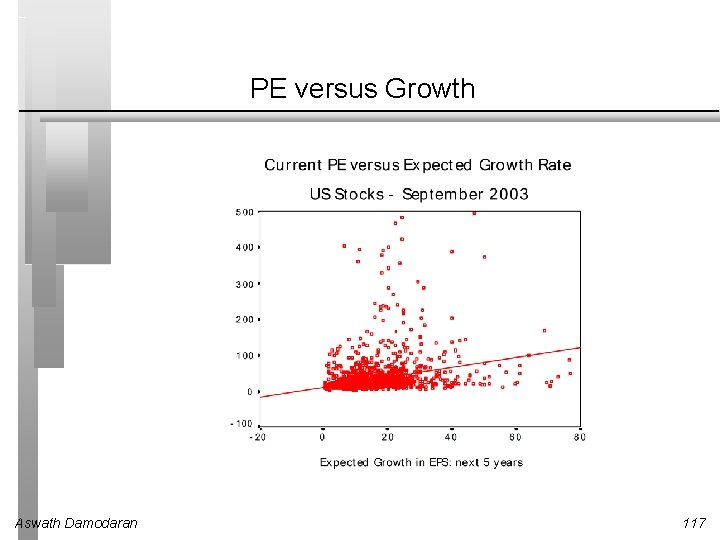PE versus Growth Aswath Damodaran 117PE Ratio: Standard Regression for US stocks September 2003 Aswath Damodaran 118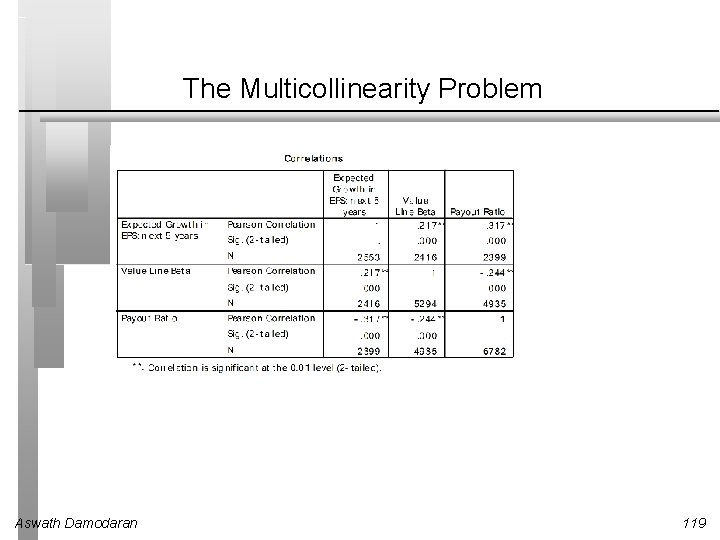The Multicollinearity Problem Aswath Damodaran 119PE Ratio without a constant - US Stocks Aswath Damodaran 120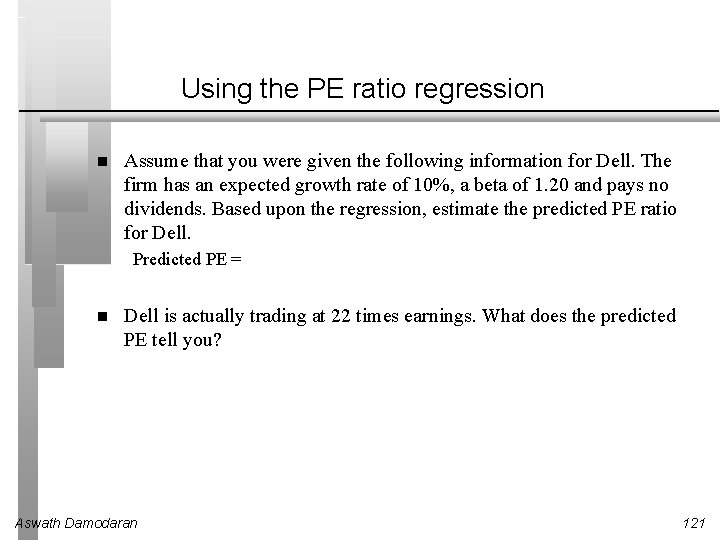Using the PE ratio regression Assume that you were given the following information for Dell. The firm has an expected growth rate of 10%, a beta of 1. 20 and pays no dividends. Based upon the regression, estimate the predicted PE ratio for Dell. Predicted PE = Dell is actually trading at 22 times earnings. What does the predicted PE tell you? Aswath Damodaran 121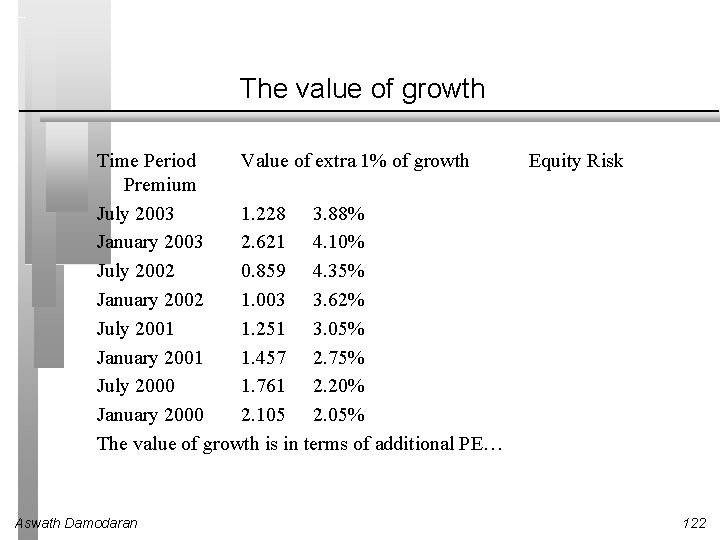The value of growth Time Period Value of extra 1% of growth Premium July 2003 1. 228 3. 88% January 2003 2. 621 4. 10% July 2002 0. 859 4. 35% January 2002 1. 003 3. 62% July 2001 1. 251 3. 05% January 2001 1. 457 2. 75% July 2000 1. 761 2. 20% January 2000 2. 105 2. 05% The value of growth is in terms of additional PE… Aswath Damodaran Equity Risk 122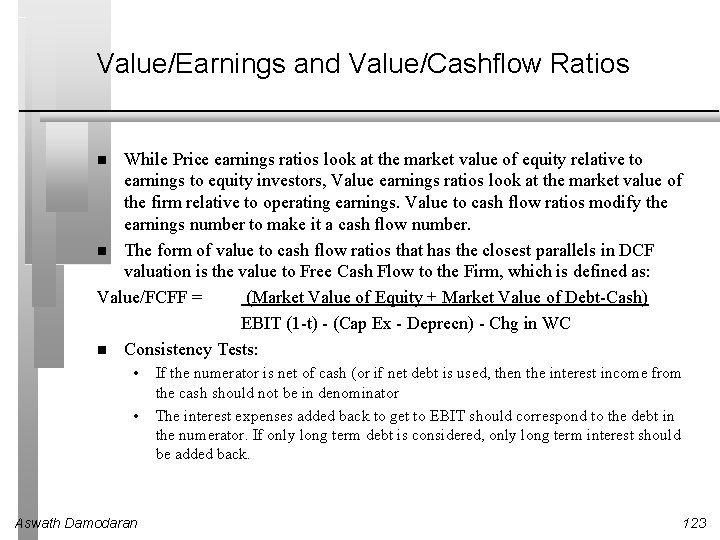Value/Earnings and Value/Cashflow Ratios While Price earnings ratios look at the market value of equity relative to earnings to equity investors, Value earnings ratios look at the market value of the firm relative to operating earnings. Value to cash flow ratios modify the earnings number to make it a cash flow number. The form of value to cash flow ratios that has the closest parallels in DCF valuation is the value to Free Cash Flow to the Firm, which is defined as: Value/FCFF = (Market Value of Equity + Market Value of Debt-Cash) EBIT (1 -t) - (Cap Ex - Deprecn) - Chg in WC Consistency Tests: • • Aswath Damodaran If the numerator is net of cash (or if net debt is used, then the interest income from the cash should not be in denominator The interest expenses added back to get to EBIT should correspond to the debt in the numerator. If only long term debt is considered, only long term interest should be added back. 123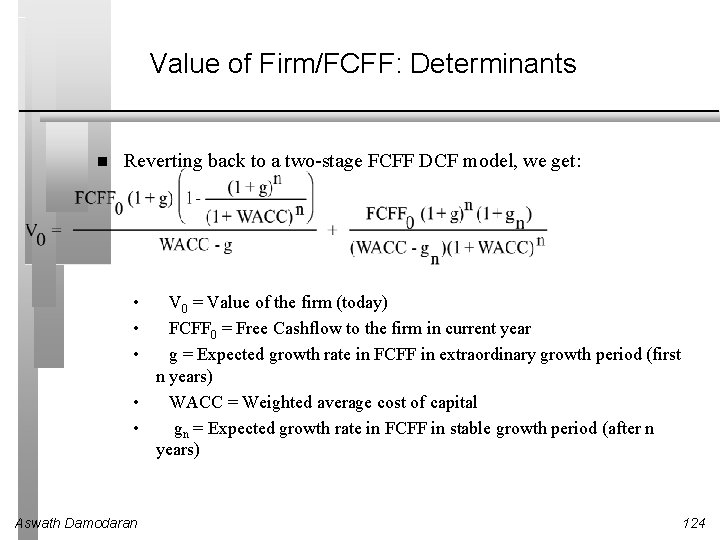Value of Firm/FCFF: Determinants Reverting back to a two-stage FCFF DCF model, we get: • • • V 0 = Value of the firm (today) FCFF 0 = Free Cashflow to the firm in current year g = Expected growth rate in FCFF in extraordinary growth period (first n years) • WACC = Weighted average cost of capital • gn = Expected growth rate in FCFF in stable growth period (after n years) Aswath Damodaran 124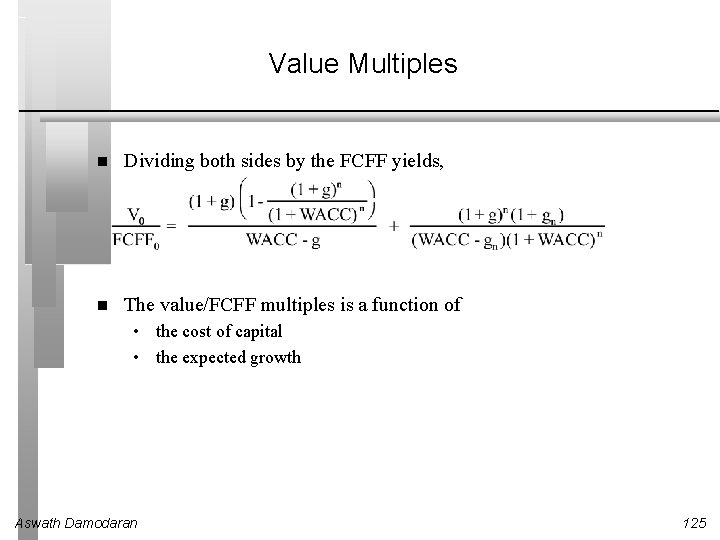Value Multiples Dividing both sides by the FCFF yields, The value/FCFF multiples is a function of • the cost of capital • the expected growth Aswath Damodaran 125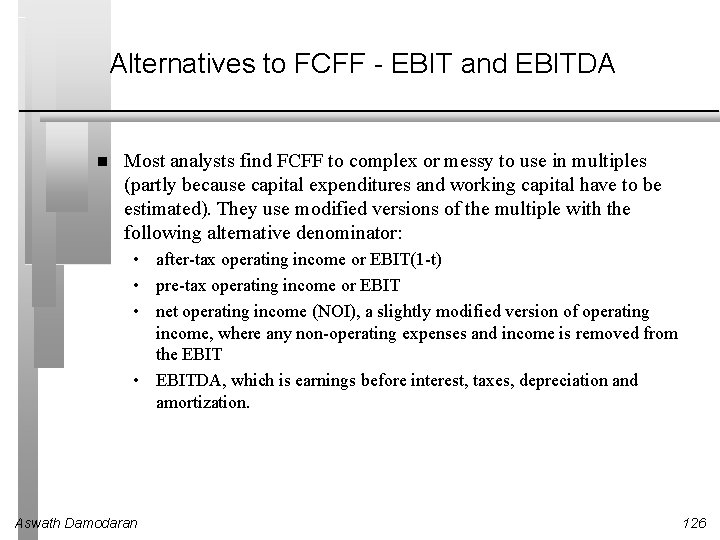Alternatives to FCFF - EBIT and EBITDA Most analysts find FCFF to complex or messy to use in multiples (partly because capital expenditures and working capital have to be estimated). They use modified versions of the multiple with the following alternative denominator: • after-tax operating income or EBIT(1 -t) • pre-tax operating income or EBIT • net operating income (NOI), a slightly modified version of operating income, where any non-operating expenses and income is removed from the EBIT • EBITDA, which is earnings before interest, taxes, depreciation and amortization. Aswath Damodaran 126Value/FCFF Multiples and the Alternatives Assume that you have computed the value of a firm, using discounted cash flow models. Rank the following multiples in the order of magnitude from lowest to highest? Value/EBIT(1 -t) Value/FCFF Value/EBITDA What assumption(s) would you need to make for the Value/EBIT(1 -t) ratio to be equal to the Value/FCFF multiple? Aswath Damodaran 127Illustration: Using Value/FCFF Approaches to value a firm: MCI Communications had earnings before interest and taxes of \$3356 million in 1994 (Its net income after taxes was \$855 million). It had capital expenditures of \$2500 million in 1994 and depreciation of \$1100 million; Working capital increased by \$250 million. It expects free cashflows to the firm to grow 15% a year for the next five years and 5% a year after that. The cost of capital is 10. 50% for the next five years and 10% after that. The company faces a tax rate of 36%. V 0 = FCFF 0 Aswath Damodaran æç (1. 15)5 ö (1. 15) è 1(1. 105)5 ø. 105 -. 15 5 (1. 15) (1. 05) = 31. 28 + 5 (. 10 -. 05)(1. 105) 128Multiple Magic In this case of MCI there is a big difference between the FCFF and short cut measures. For instance the following table illustrates the appropriate multiple using short cut measures, and the amount you would overpay by if you used the FCFF multiple. Free Cash Flow to the Firm = EBIT (1 -t) - Net Cap Ex - Change in Working Capital = 3356 (1 - 0. 36) + 1100 - 250 = \$ 498 million \$ Value Correct Multiple FCFF \$498 31. 28382355 EBIT (1 -t) \$2, 148 7. 251163362 EBIT \$ 3, 356 4. 640744552 EBITDA \$4, 456 3. 49513885 Aswath Damodaran 129Reasons for Increased Use of Value/EBITDA 1. The multiple can be computed even for firms that are reporting net losses, since earnings before interest, taxes and depreciation are usually positive. 2. For firms in certain industries, such as cellular, which require a substantial investment in infrastructure and long gestation periods, this multiple seems to be more appropriate than the price/earnings ratio. 3. In leveraged buyouts, where the key factor is cash generated by the firm prior to all discretionary expenditures, the EBITDA is the measure of cash flows from operations that can be used to support debt payment at least in the short term. 4. By looking at cashflows prior to capital expenditures, it may provide a better estimate of “optimal value”, especially if the capital expenditures are unwise or earn substandard returns. 5. By looking at the value of the firm and cashflows to the firm it allows for comparisons across firms with different financial leverage. Aswath Damodaran 130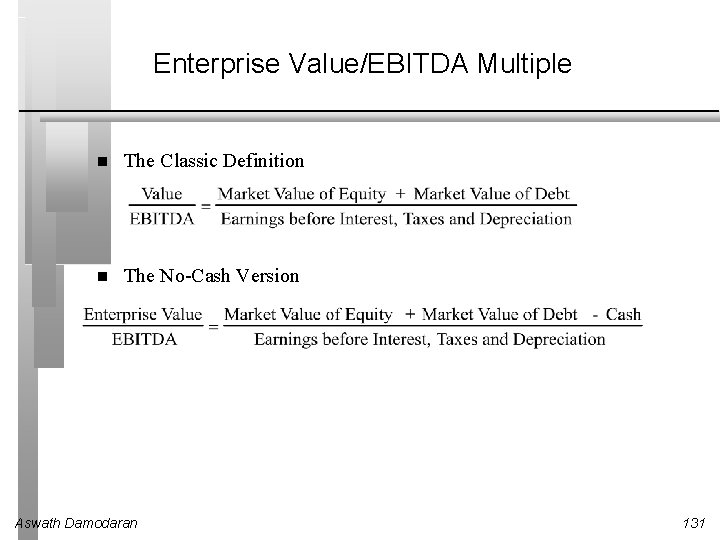Enterprise Value/EBITDA Multiple The Classic Definition The No-Cash Version Aswath Damodaran 131Value/EBITDA Distribution - US in September 2003 Aswath Damodaran 132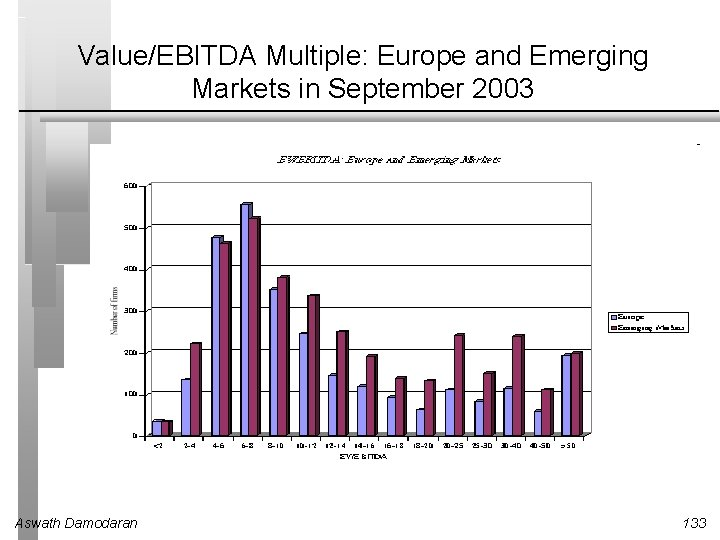Value/EBITDA Multiple: Europe and Emerging Markets in September 2003 Aswath Damodaran 133The Determinants of Value/EBITDA Multiples: Linkage to DCF Valuation Firm value can be written as: The numerator can be written as follows: FCFF Aswath Damodaran = EBIT (1 -t) - (Cex - Depr) - Working Capital = (EBITDA - Depr) (1 -t) - (Cex - Depr) - Working Capital = EBITDA (1 -t) + Depr (t) - Cex - Working Capital 134From Firm Value to EBITDA Multiples Now the Value of the firm can be rewritten as, Dividing both sides of the equation by EBITDA, Aswath Damodaran 135A Simple Example Consider a firm with the following characteristics: • • • Aswath Damodaran Tax Rate = 36% Capital Expenditures/EBITDA = 30% Depreciation/EBITDA = 20% Cost of Capital = 10% The firm has no working capital requirements The firm is in stable growth and is expected to grow 5% a year forever. 136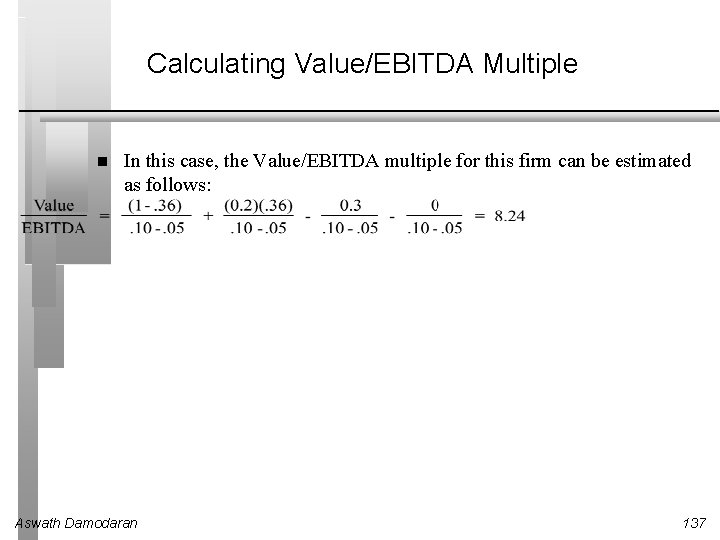Calculating Value/EBITDA Multiple In this case, the Value/EBITDA multiple for this firm can be estimated as follows: Aswath Damodaran 137Value/EBITDA Multiples and Taxes Aswath Damodaran 138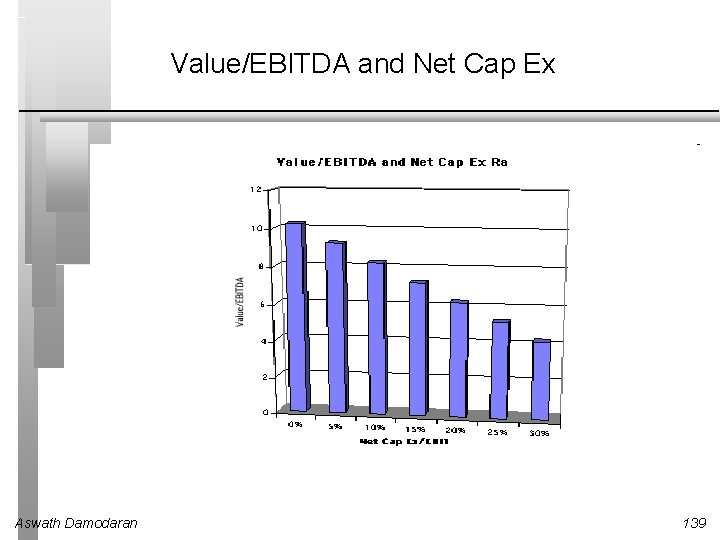Value/EBITDA and Net Cap Ex Aswath Damodaran 139Value/EBITDA Multiples and Return on Capital Aswath Damodaran 140Value/EBITDA Multiple: Trucking Companies Aswath Damodaran 141A Test on EBITDA Ryder System looks very cheap on a Value/EBITDA multiple basis, relative to the rest of the sector. What explanation (other than misvaluation) might there be for this difference? Aswath Damodaran 142US Market: Cross Sectional Regression September 2003 Aswath Damodaran 143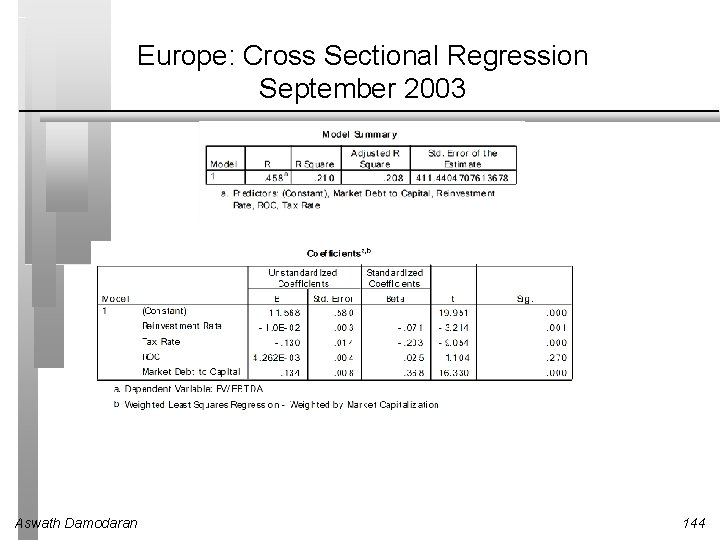Europe: Cross Sectional Regression September 2003 Aswath Damodaran 144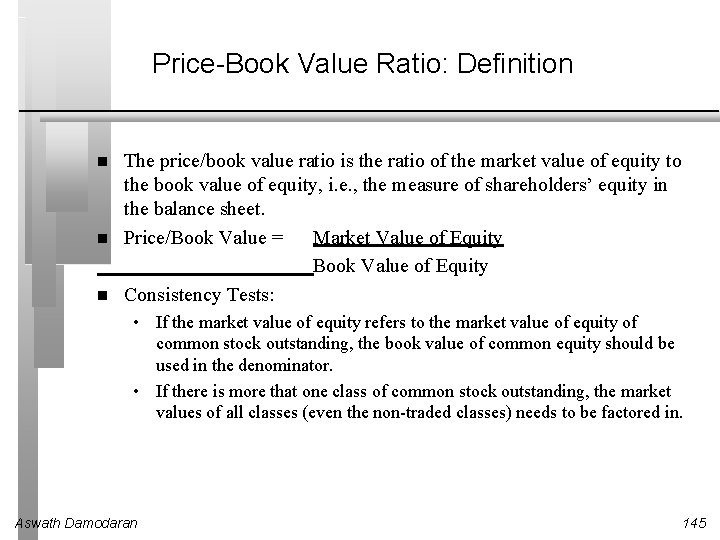Price-Book Value Ratio: Definition The price/book value ratio is the ratio of the market value of equity to the book value of equity, i. e. , the measure of shareholders’ equity in the balance sheet. Price/Book Value = Market Value of Equity Book Value of Equity Consistency Tests: • If the market value of equity refers to the market value of equity of common stock outstanding, the book value of common equity should be used in the denominator. • If there is more that one class of common stock outstanding, the market values of all classes (even the non-traded classes) needs to be factored in. Aswath Damodaran 145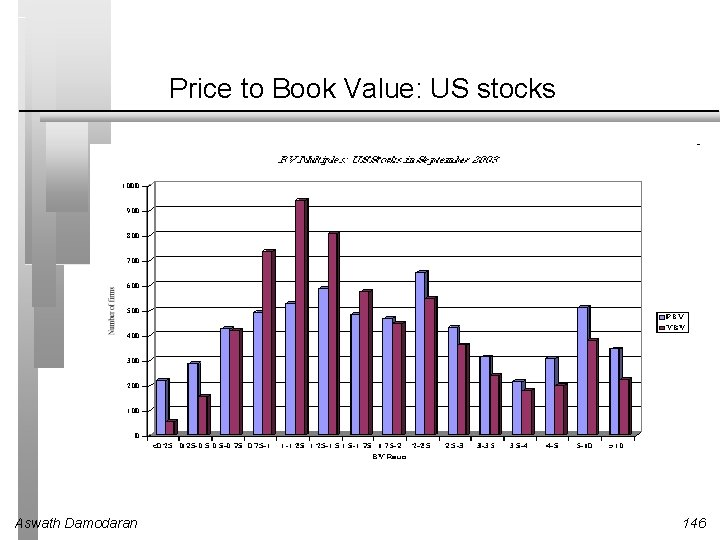Price to Book Value: US stocks Aswath Damodaran 146Price to Book: Europe and Emerging Markets Aswath Damodaran 147Price Book Value Ratio: Stable Growth Firm Going back to a simple dividend discount model, Defining the return on equity (ROE) = EPS 0 / Book Value of Equity, the value of equity can be written as: If the return on equity is based upon expected earnings in the next time period, this can be simplified to, Aswath Damodaran 148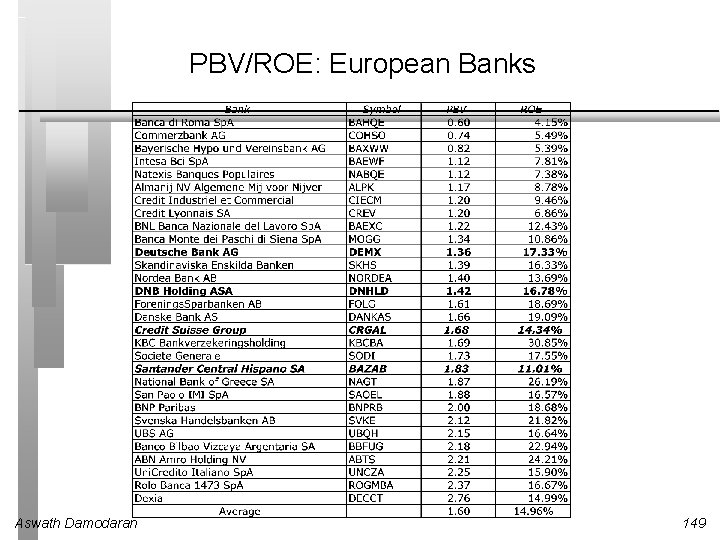PBV/ROE: European Banks Aswath Damodaran 149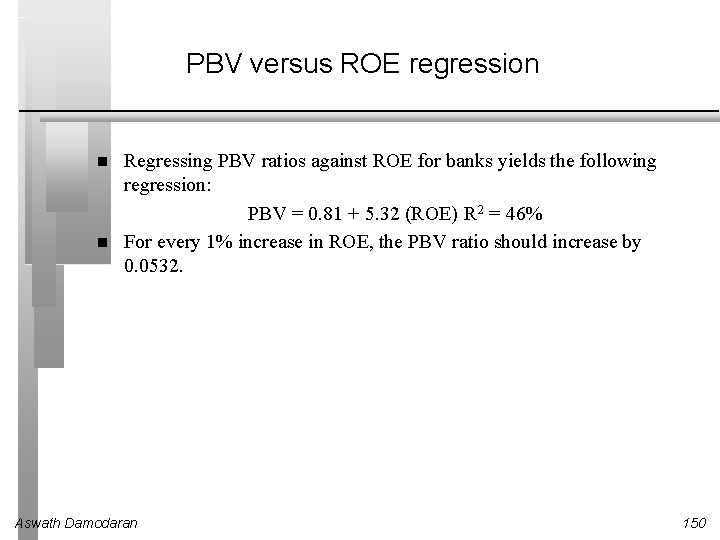PBV versus ROE regression Regressing PBV ratios against ROE for banks yields the following regression: PBV = 0. 81 + 5. 32 (ROE) R 2 = 46% For every 1% increase in ROE, the PBV ratio should increase by 0. 0532. Aswath Damodaran 150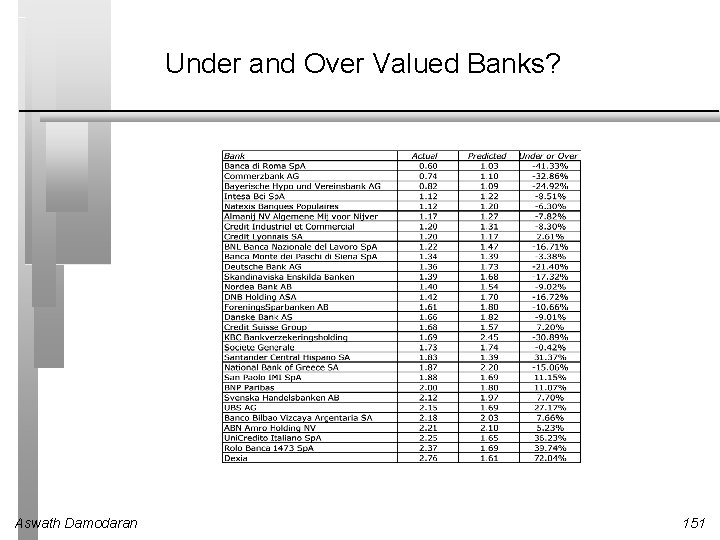Under and Over Valued Banks? Aswath Damodaran 151Looking for undervalued securities - PBV Ratios and ROE Given the relationship between price-book value ratios and returns on equity, it is not surprising to see firms which have high returns on equity selling for well above book value and firms which have low returns on equity selling at or below book value. The firms which should draw attention from investors are those which provide mismatches of price-book value ratios and returns on equity low P/BV ratios and high ROE or high P/BV ratios and low ROE. Aswath Damodaran 152The Valuation Matrix Aswath Damodaran 153Price to Book vs ROE: Largest Market Cap Firms in the United States: September 2003 Aswath Damodaran 154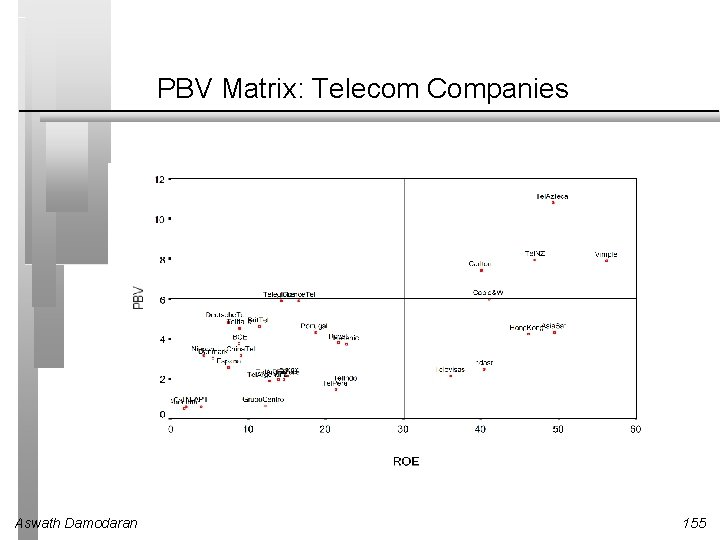PBV Matrix: Telecom Companies Aswath Damodaran 155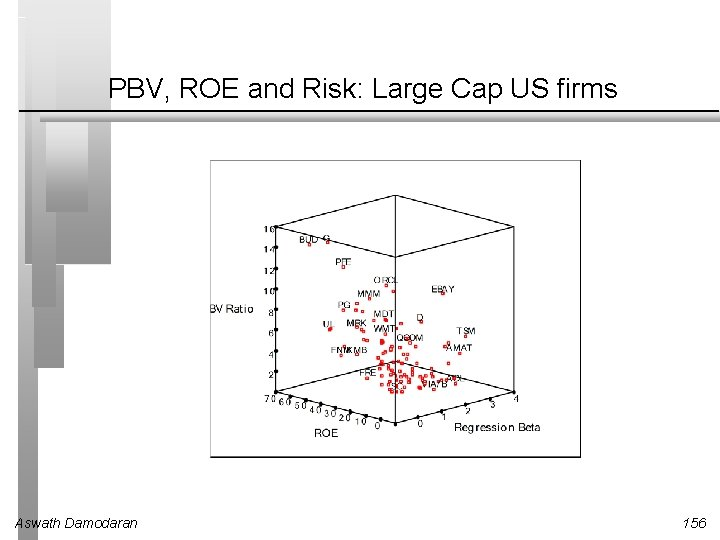PBV, ROE and Risk: Large Cap US firms Aswath Damodaran 156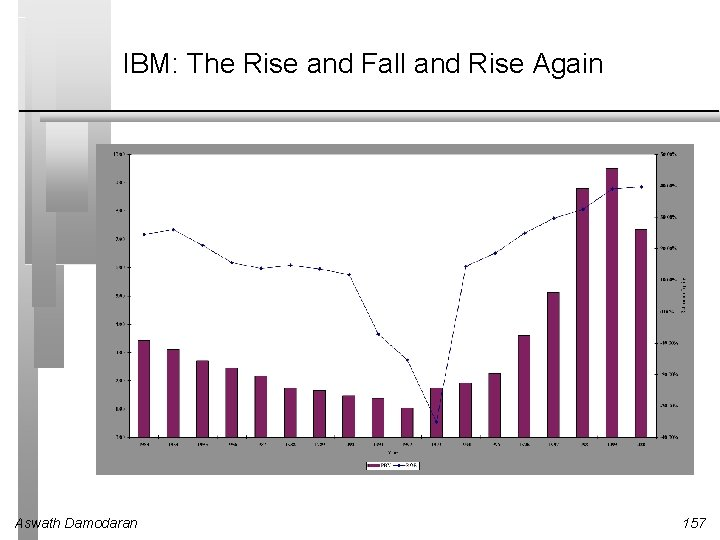IBM: The Rise and Fall and Rise Again Aswath Damodaran 157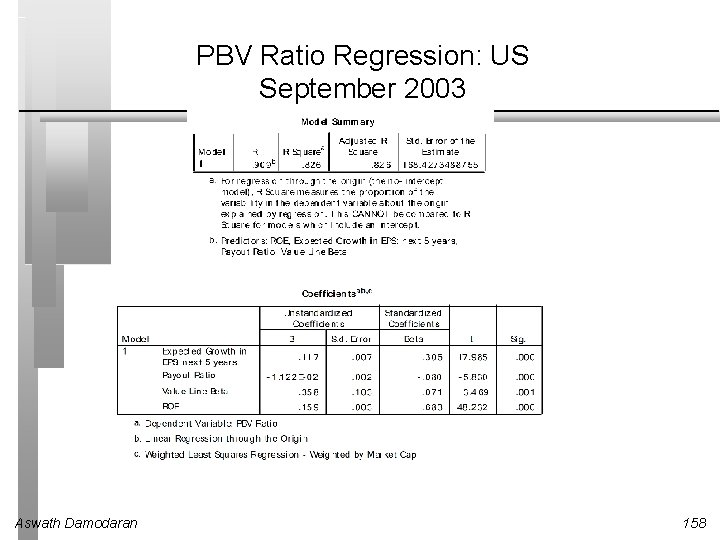PBV Ratio Regression: US September 2003 Aswath Damodaran 158PBV Ratio Regression- Europe September 2003 Aswath Damodaran 159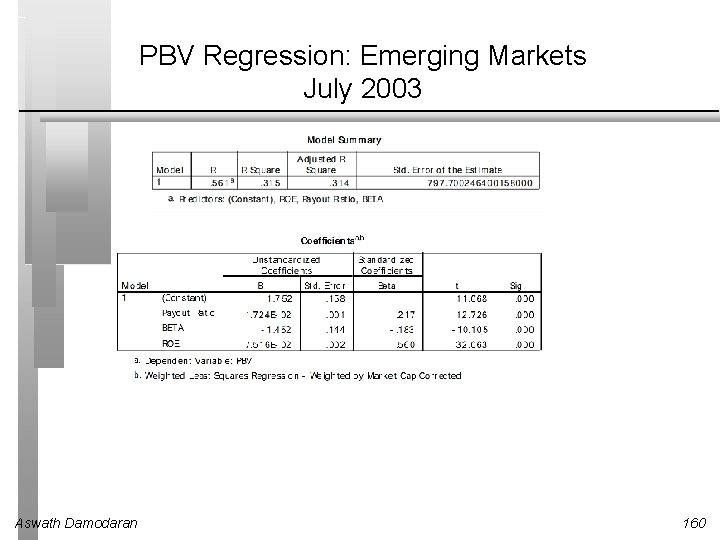PBV Regression: Emerging Markets July 2003 Aswath Damodaran 160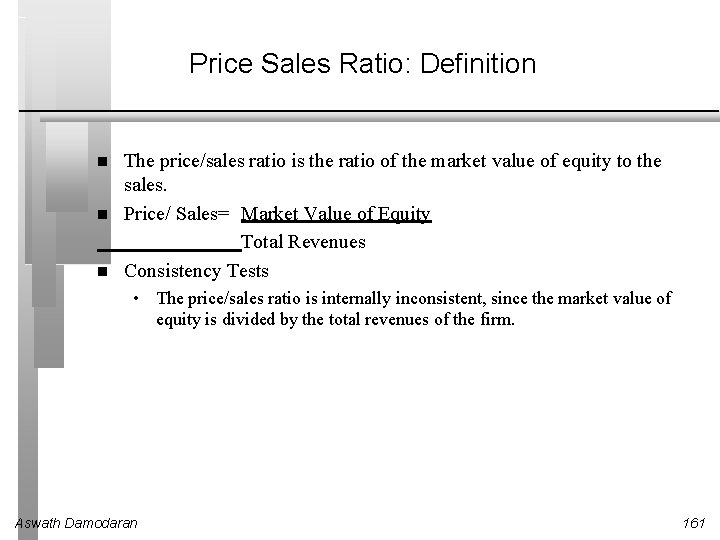Price Sales Ratio: Definition The price/sales ratio is the ratio of the market value of equity to the sales. Price/ Sales= Market Value of Equity Total Revenues Consistency Tests • The price/sales ratio is internally inconsistent, since the market value of equity is divided by the total revenues of the firm. Aswath Damodaran 161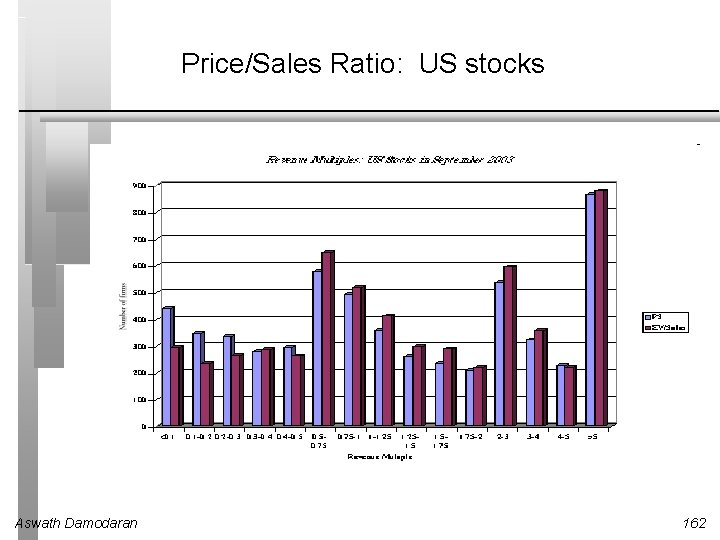Price/Sales Ratio: US stocks Aswath Damodaran 162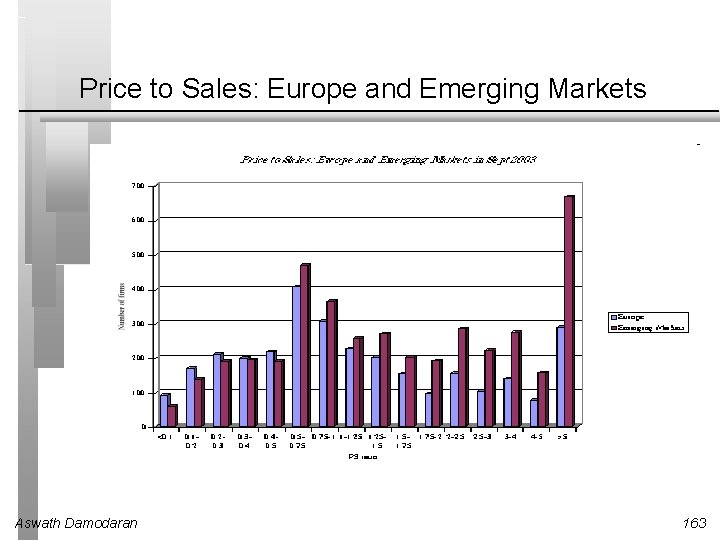Price to Sales: Europe and Emerging Markets Aswath Damodaran 163Price/Sales Ratio: Determinants The price/sales ratio of a stable growth firm can be estimated beginning with a 2 -stage equity valuation model: Dividing both sides by the sales per share: Aswath Damodaran 164PS/Margins: European Retailers - September 2003 Aswath Damodaran 165Regression Results: PS Ratios and Margins Regressing PS ratios against net margins, PS = -. 39 + 0. 6548 (Net Margin) R 2 = 43. 5% Thus, a 1% increase in the margin results in an increase of 0. 6548 in the price sales ratios. The regression also allows us to get predicted PS ratios for these firms Aswath Damodaran 166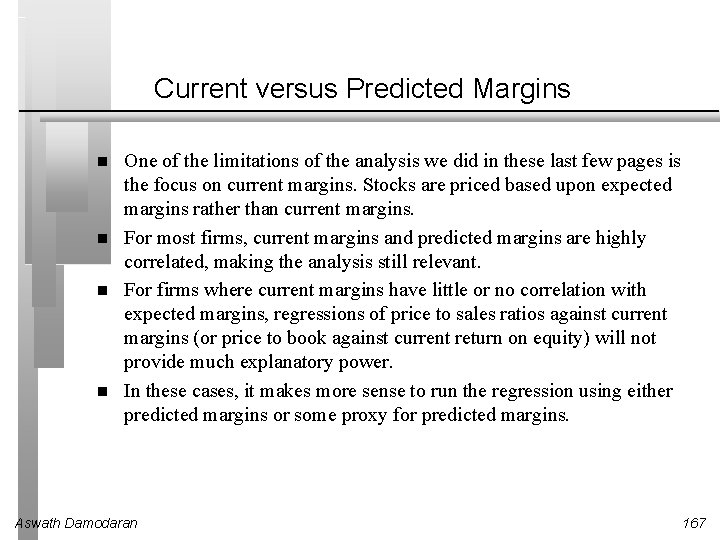Current versus Predicted Margins One of the limitations of the analysis we did in these last few pages is the focus on current margins. Stocks are priced based upon expected margins rather than current margins. For most firms, current margins and predicted margins are highly correlated, making the analysis still relevant. For firms where current margins have little or no correlation with expected margins, regressions of price to sales ratios against current margins (or price to book against current return on equity) will not provide much explanatory power. In these cases, it makes more sense to run the regression using either predicted margins or some proxy for predicted margins. Aswath Damodaran 167A Case Study: The Internet Stocks Aswath Damodaran 168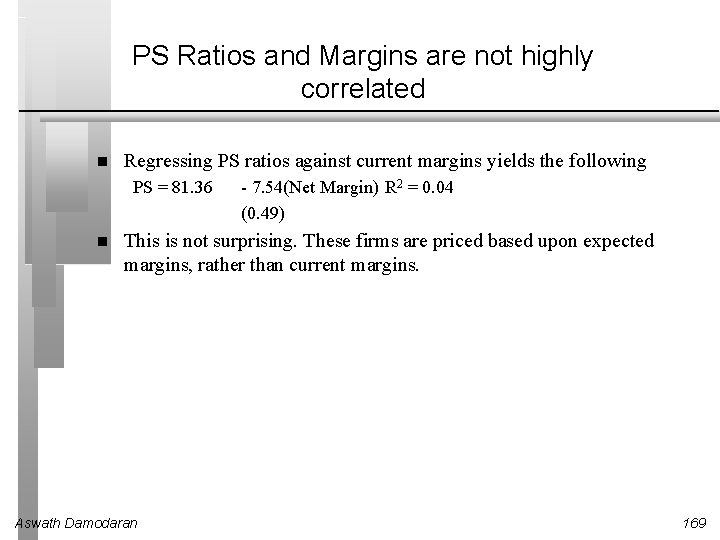PS Ratios and Margins are not highly correlated Regressing PS ratios against current margins yields the following PS = 81. 36 - 7. 54(Net Margin) R 2 = 0. 04 (0. 49) This is not surprising. These firms are priced based upon expected margins, rather than current margins. Aswath Damodaran 169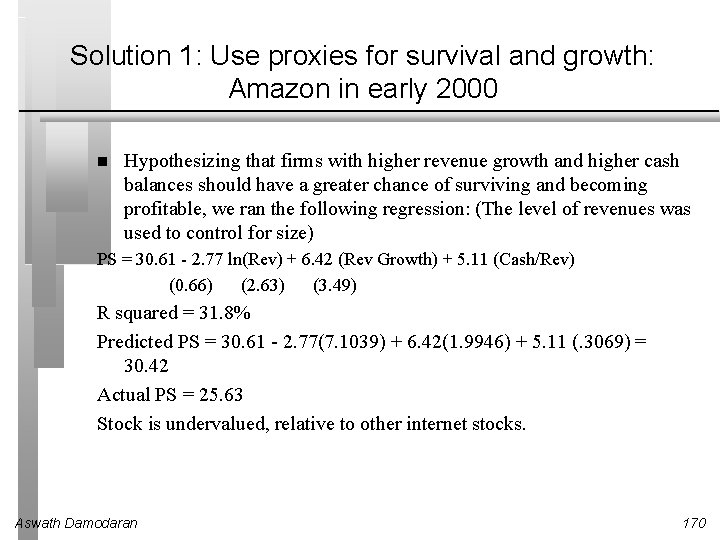Solution 1: Use proxies for survival and growth: Amazon in early 2000 Hypothesizing that firms with higher revenue growth and higher cash balances should have a greater chance of surviving and becoming profitable, we ran the following regression: (The level of revenues was used to control for size) PS = 30. 61 - 2. 77 ln(Rev) + 6. 42 (Rev Growth) + 5. 11 (Cash/Rev) (0. 66) (2. 63) (3. 49) R squared = 31. 8% Predicted PS = 30. 61 - 2. 77(7. 1039) + 6. 42(1. 9946) + 5. 11 (. 3069) = 30. 42 Actual PS = 25. 63 Stock is undervalued, relative to other internet stocks. Aswath Damodaran 170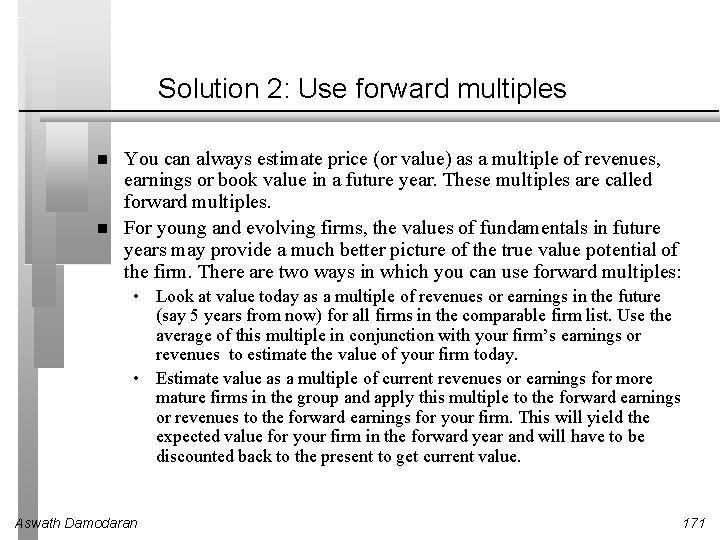Solution 2: Use forward multiples You can always estimate price (or value) as a multiple of revenues, earnings or book value in a future year. These multiples are called forward multiples. For young and evolving firms, the values of fundamentals in future years may provide a much better picture of the true value potential of the firm. There are two ways in which you can use forward multiples: • Look at value today as a multiple of revenues or earnings in the future (say 5 years from now) for all firms in the comparable firm list. Use the average of this multiple in conjunction with your firm’s earnings or revenues to estimate the value of your firm today. • Estimate value as a multiple of current revenues or earnings for more mature firms in the group and apply this multiple to the forward earnings or revenues to the forward earnings for your firm. This will yield the expected value for your firm in the forward year and will have to be discounted back to the present to get current value. Aswath Damodaran 171An Example of Forward Multiples: Global Crossing lost \$1. 9 billion in 2001 and is expected to continue to lose money for the next 3 years. In a discounted cashflow valuation (see notes on DCF valuation) of Global Crossing, we estimated an expected EBITDA for Global Crossing in five years of \$ 1, 371 million. The average enterprise value/ EBITDA multiple for healthy telecomm firms is 7. 2 currently. Applying this multiple to Global Crossing’s EBITDA in year 5, yields a value in year 5 of • Enterprise Value in year 5 = 1371 * 7. 2 = \$9, 871 million • Enterprise Value today = \$ 9, 871 million/ 1. 1385 = \$5, 172 million (The cost of capital for Global Crossing is 13. 80%) • The probability that Global Crossing will not make it as a going concern is 77% and the distress sale value is only a \$ 1 billion (1/2 of book value of assets). • Adjusted Enterprise value = 5172 *. 23 + 1000 (. 77) = 1, 960 million Aswath Damodaran 172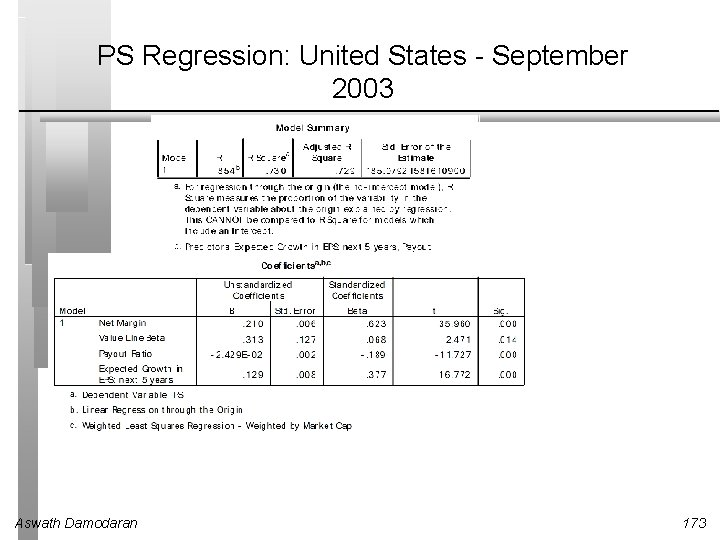PS Regression: United States - September 2003 Aswath Damodaran 173PS Regression: Europe in September 2003 Aswath Damodaran 174PS Regression in Emerging Markets - July 2003 Aswath Damodaran 175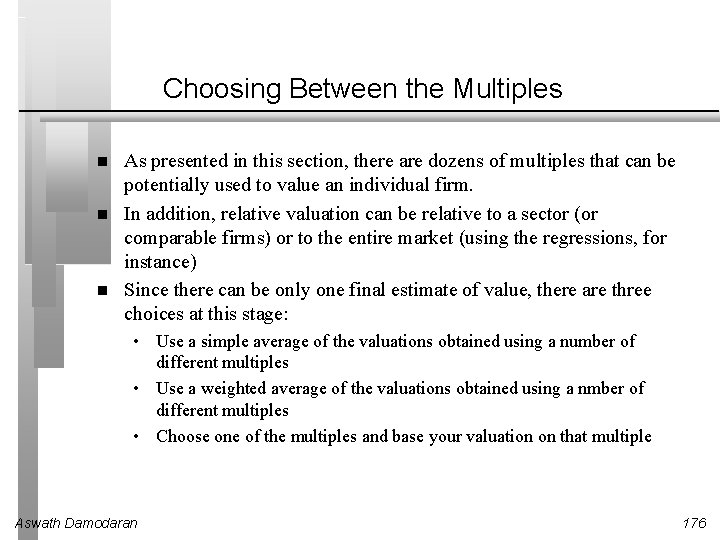Choosing Between the Multiples As presented in this section, there are dozens of multiples that can be potentially used to value an individual firm. In addition, relative valuation can be relative to a sector (or comparable firms) or to the entire market (using the regressions, for instance) Since there can be only one final estimate of value, there are three choices at this stage: • Use a simple average of the valuations obtained using a number of different multiples • Use a weighted average of the valuations obtained using a nmber of different multiples • Choose one of the multiples and base your valuation on that multiple Aswath Damodaran 176Picking one Multiple This is usually the best way to approach this issue. While a range of values can be obtained from a number of multiples, the “best estimate” value is obtained using one multiple. The multiple that is used can be chosen in one of two ways: • Use the multiple that best fits your objective. Thus, if you want the company to be undervalued, you pick the multiple that yields the highest value. • Use the multiple that has the highest R-squared in the sector when regressed against fundamentals. Thus, if you have tried PE, PBV, PS, etc. and run regressions of these multiples against fundamentals, use the multiple that works best at explaining differences across firms in that sector. • Use the multiple that seems to make the most sense for that sector, given how value is measured and created. Aswath Damodaran 177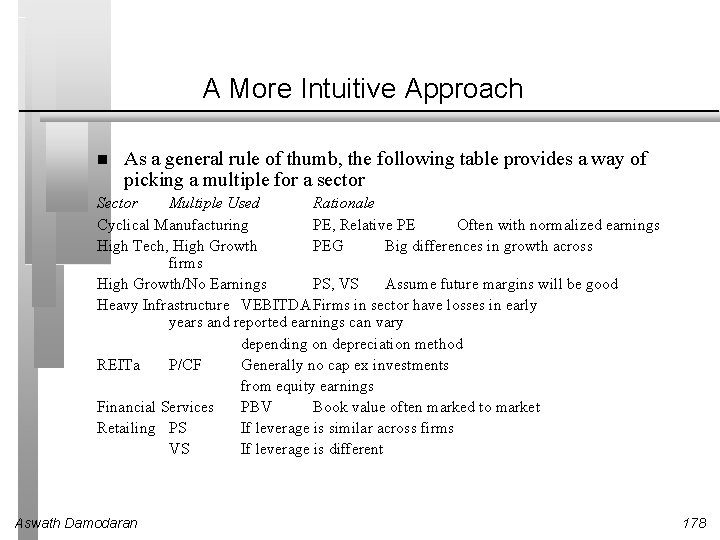A More Intuitive Approach As a general rule of thumb, the following table provides a way of picking a multiple for a sector Sector Multiple Used Rationale Cyclical Manufacturing PE, Relative PE Often with normalized earnings High Tech, High Growth PEG Big differences in growth across firms High Growth/No Earnings PS, VS Assume future margins will be good Heavy Infrastructure VEBITDA Firms in sector have losses in early years and reported earnings can vary depending on depreciation method REITa P/CF Generally no cap ex investments from equity earnings Financial Services PBV Book value often marked to market Retailing PS If leverage is similar across firms VS If leverage is different Aswath Damodaran 178Reviewing: The Four Steps to Understanding Multiples Define the multiple • Check for consistency • Make sure that they are estimated uniformally Describe the multiple • Multiples have skewed distributions: The averages are seldom good indicators of typical multiples • Check for bias, if the multiple cannot be estimated Analyze the multiple • Identify the companion variable that drives the multiple • Examine the nature of the relationship Apply the multiple Aswath Damodaran 179